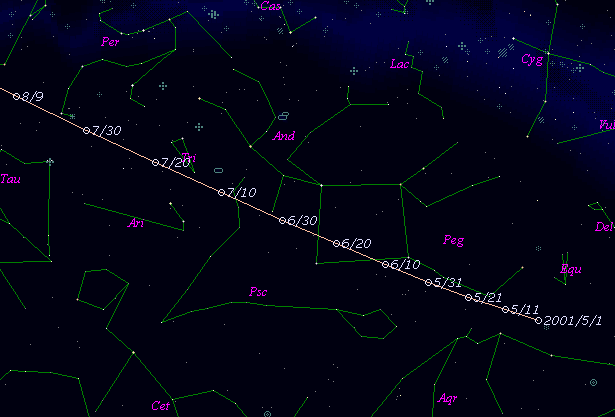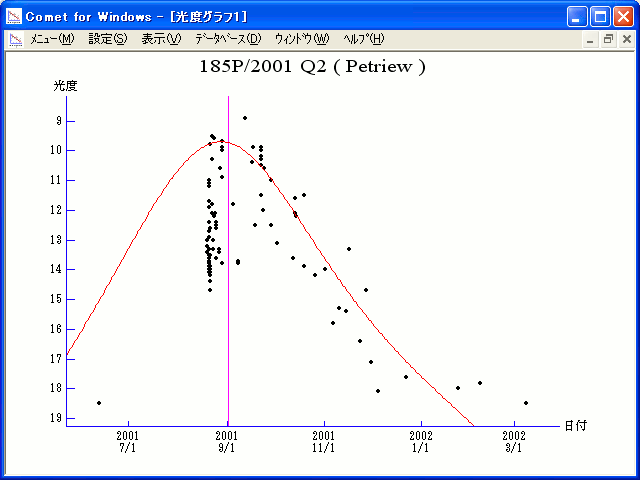# \$B%Z%H%j%e!

185P/Petriew (2001)

 English version Home page Updated on June 24, 2012###\$B%W%m%U%#!<%k(B

 \$BId9f(B 185P/2001 Q2 \$BH/8+F|(B 2001\$BG/(B8\$B7n(B18\$BF|(B \$BH/8+8wEY(B 11.0\$BEy(B \$BH/8+ V. A. Petriew (Cyprus Hills, SK)

###\$B50F;MWAG(B

```                    Epoch = 2001 Sept. 8.0 TT
T = 2001 Sept. 1.9221 TT         Peri. = 181.9012
e = 0.696237                     Node  = 214.1070  2000.0
q = 0.945691 AU                  Incl. =  13.9444
a =  3.113256 AU    n = 0.1794246    P =   5.49 years
```

###\$B@1?^(B###\$B8wEYJQ2=(B

```        m1 = 10.5 + 5 log\$B&\$(B + 25.0 log r
```##### \$B50F;MWAG\$O(B Dr. Brian G. Marsden \$B\$N7W;;\$K\$h\$k\$b\$N\$G!"(B IAUC 8796 \$B\$K7G:\\$5\$l\$?\$b\$N\$G\$9!#(B \$B@1?^\$O(B StellaNavigator Ver.2.0 for Windows (\$B%"%9%H%m%"!<%D(B \$BJTCx(B / \$B%"%9%-!<=PHG6I4)(B) \$B\$G:n@.\$7\$?\$b\$N\$G\$9!#(B \$B8wEY%0%i%U\$O(BComet for Windows\$B\$G:n@.\$7\$?\$b\$N\$G\$9!#(BCopyright(C) Seiichi Yoshida (comet@aerith.net). All rights reserved.